Here, we’ll describe how to produce a matrix of scatter plots. This is useful to visualize correlation of small data sets. The R base function pairs() can be used.

``head(iris)``
``````##   Sepal.Length Sepal.Width Petal.Length Petal.Width Species
## 1          5.1         3.5          1.4         0.2  setosa
## 2          4.9         3.0          1.4         0.2  setosa
## 3          4.7         3.2          1.3         0.2  setosa
## 4          4.6         3.1          1.5         0.2  setosa
## 5          5.0         3.6          1.4         0.2  setosa
## 6          5.4         3.9          1.7         0.4  setosa``````
``pairs(iris[,1:4], pch = 19)``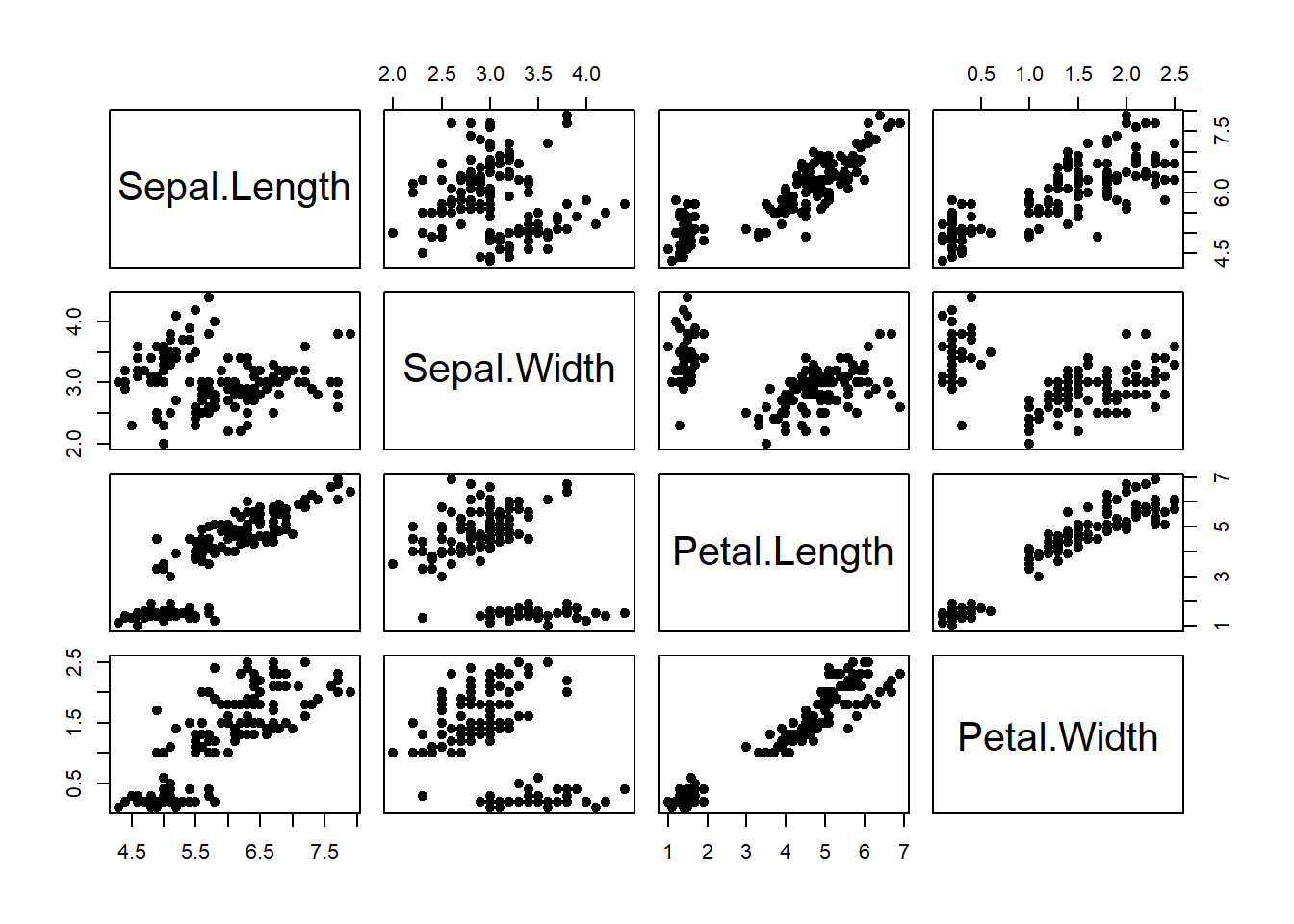``````# Show only upper triangle:

pairs(iris[,1:4], pch = 19, lower.panel = NULL)``````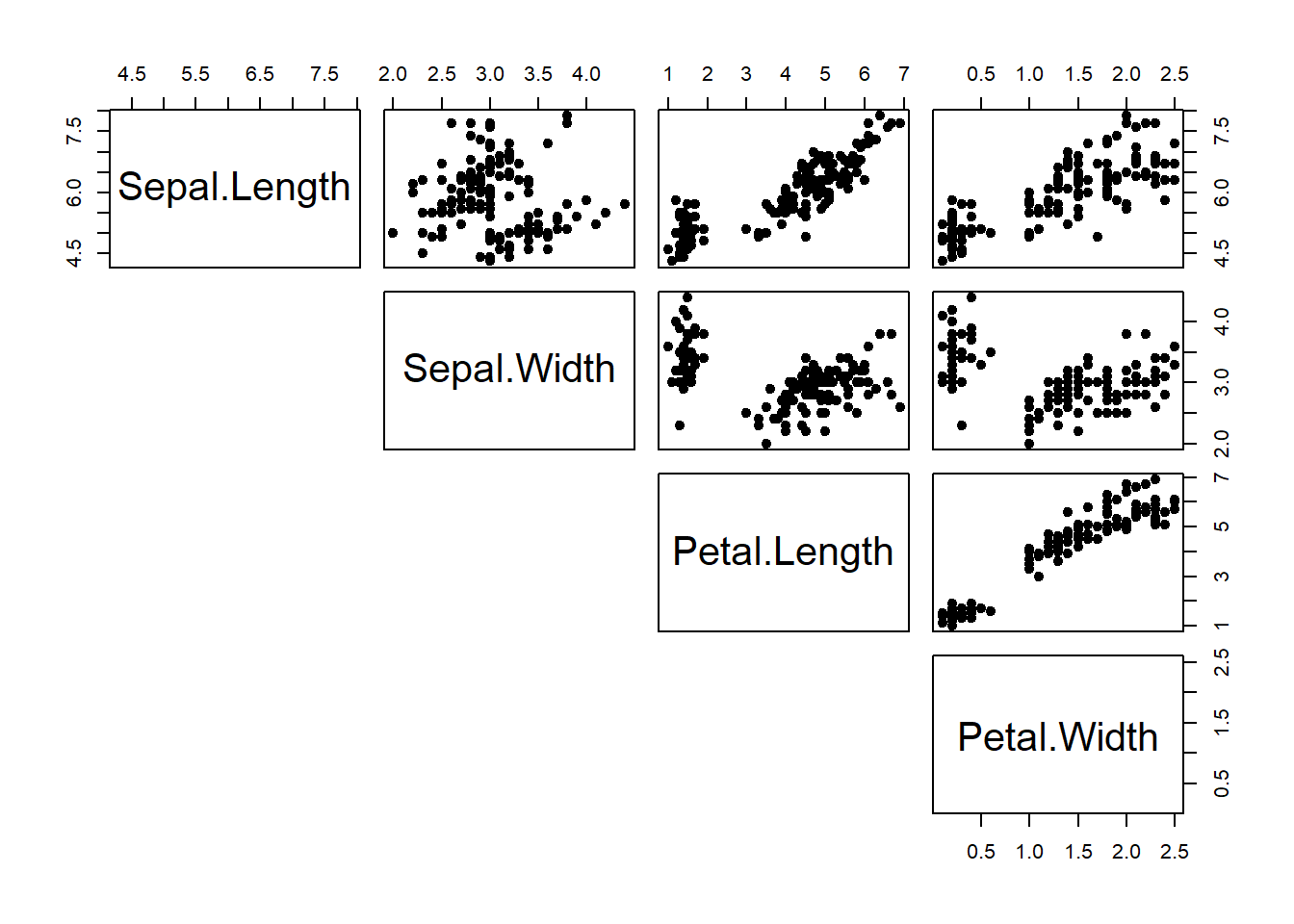Color Group by Points

``````my_cols <- c("#00AFBB", "#E7B800", "#FC4E07")
pairs(iris[,1:4], pch = 19,  cex = 0.5,
col = my_cols[iris\$Species],
lower.panel=NULL)``````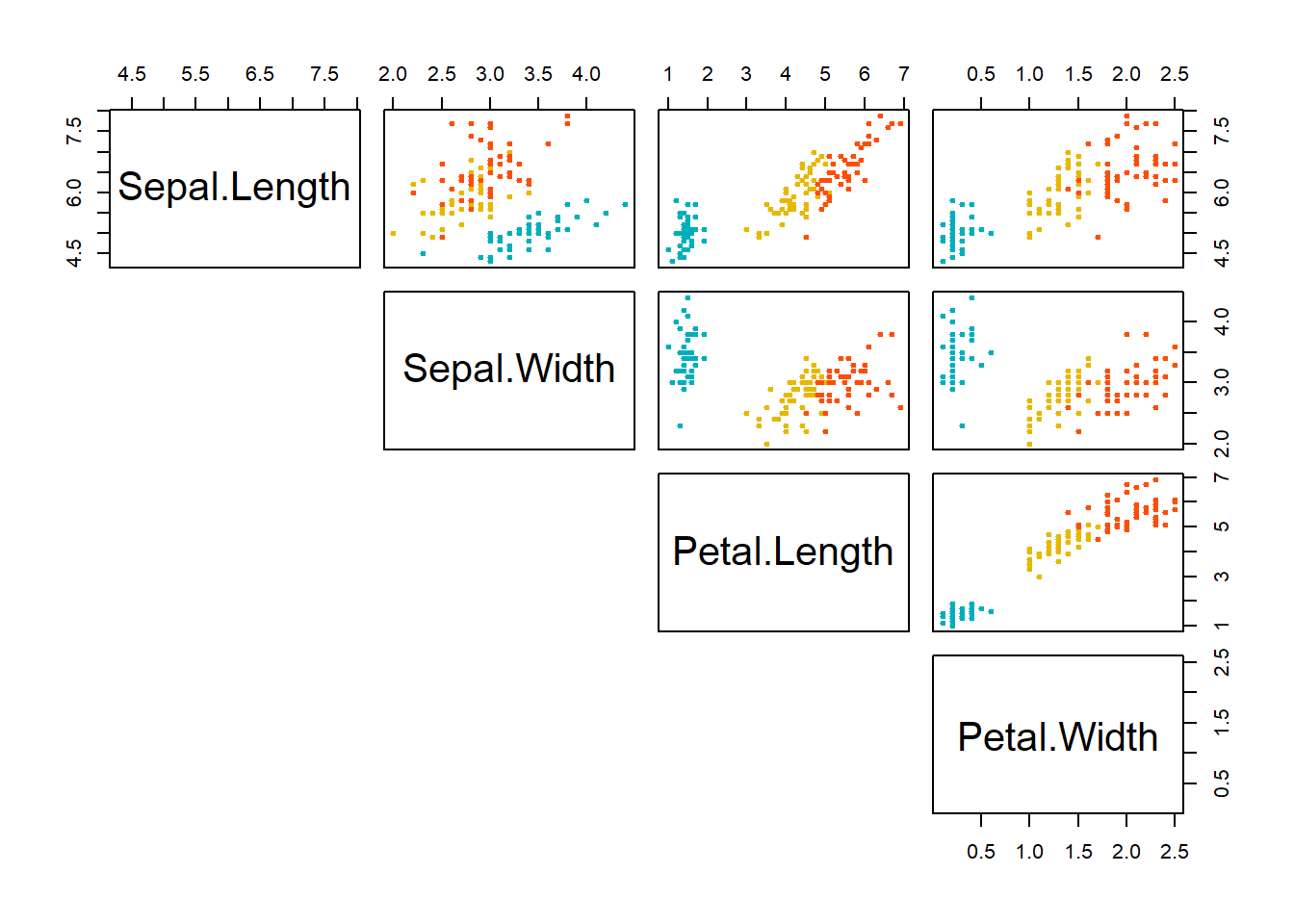``````# Correlation panel
panel.cor <- function(x, y){
usr <- par("usr"); on.exit(par(usr))
par(usr = c(0, 1, 0, 1))
r <- round(cor(x, y), digits=2)
txt <- paste0("R = ", r)
cex.cor <- 0.8/strwidth(txt)
text(0.5, 0.5, txt, cex = cex.cor * r)
}
# Customize upper panel
upper.panel<-function(x, y){
points(x,y, pch = 19, col = my_cols[iris\$Species])
}
# Create the plots
pairs(iris[,1:4],
lower.panel = panel.cor,
upper.panel = upper.panel)``````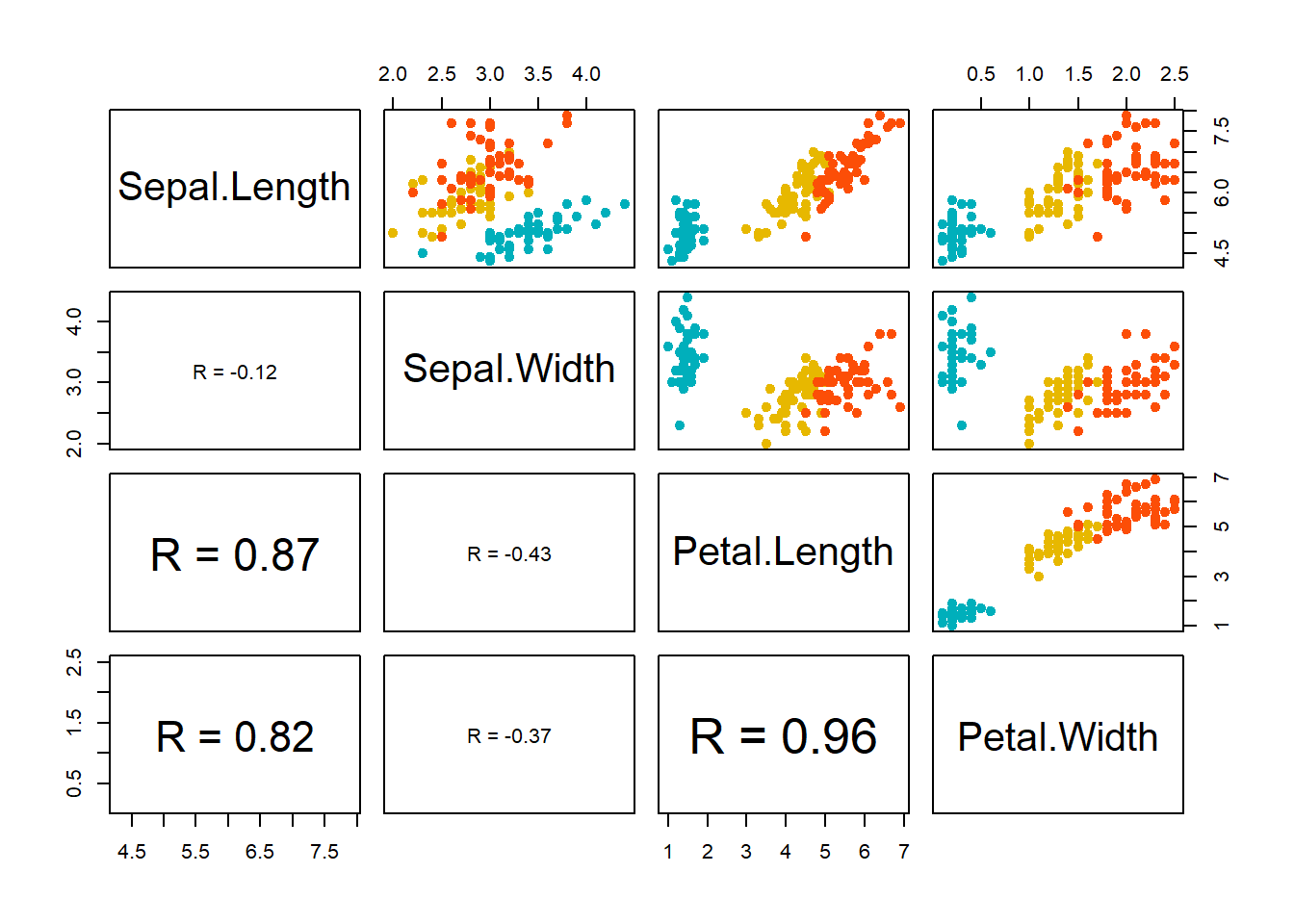Add correlation on the scatter plot

``````# Customize upper panel
upper.panel<-function(x, y){
points(x,y, pch=19, col=c("red", "green3", "blue")[iris\$Species])
r <- round(cor(x, y), digits=2)
txt <- paste0("R = ", r)
usr <- par("usr"); on.exit(par(usr))
par(usr = c(0, 1, 0, 1))
text(0.5, 0.9, txt)
}
pairs(iris[,1:4], lower.panel = NULL,
upper.panel = upper.panel)``````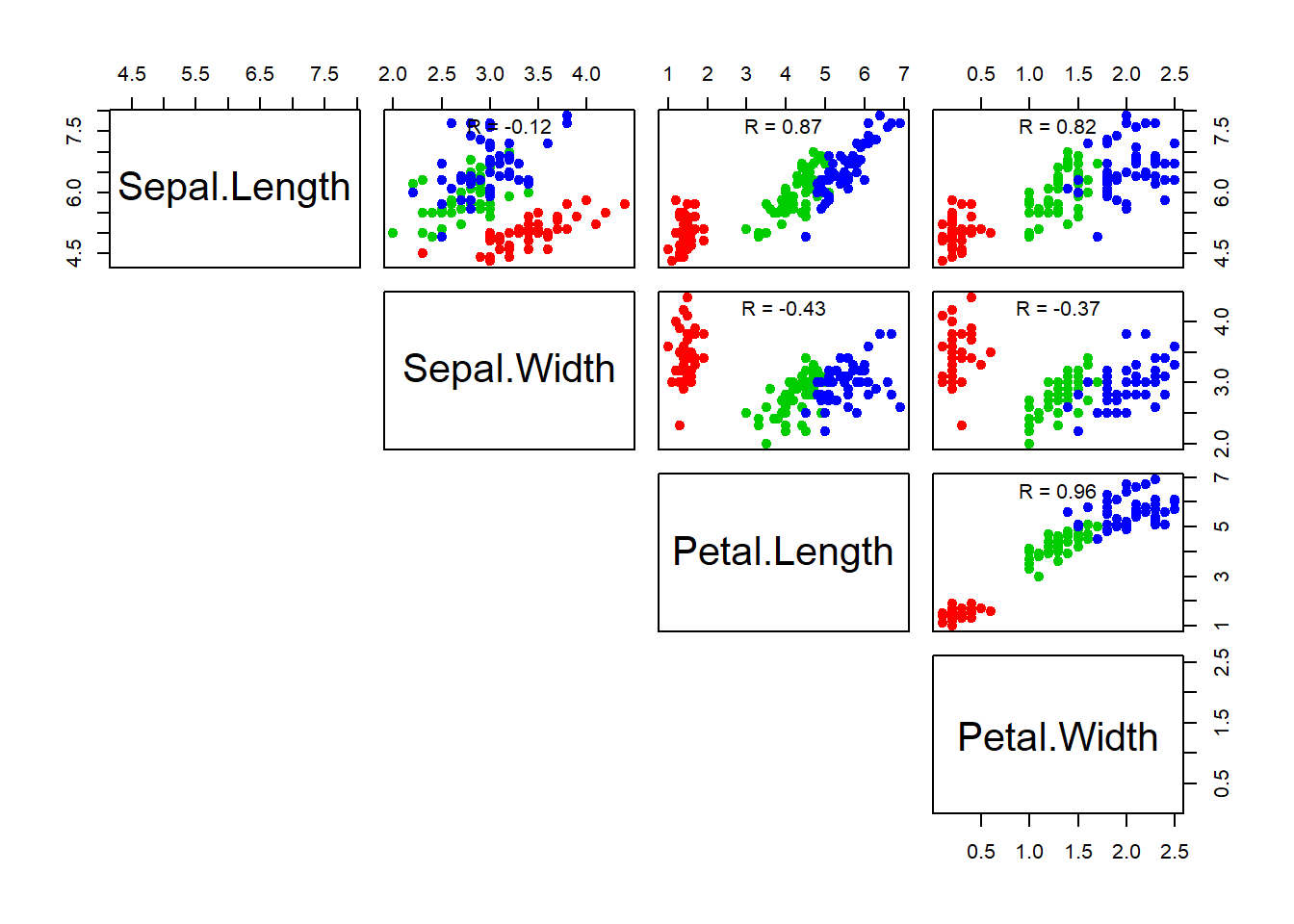R Documentation

pairs(x, …) # S3 method for formula pairs(formula, data = NULL, …, subset, na.action = stats::na.pass)

# S3 method for default

pairs(x, labels, panel = points, …, horInd = 1:nc, verInd = 1:nc, lower.panel = panel, upper.panel = panel, diag.panel = NULL, text.panel = textPanel, label.pos = 0.5 + has.diag/3, line.main = 3, cex.labels = NULL, font.labels = 1, row1attop = TRUE, gap = 1, log = "", horOdd = !row1attop, verOdd = !row1attop)

``  Arguments``

x the coordinates of points given as numeric columns of a matrix or data frame. Logical and factor columns are converted to numeric in the same way that data.matrix does.

formula a formula, such as ~ x + y + z. Each term will give a separate variable in the pairs plot, so terms should be numeric vectors. (A response will be interpreted as another variable, but not treated specially, so it is confusing to use one.)

data a data.frame (or list) from which the variables in formula should be taken.

subset an optional vector specifying a subset of observations to be used for plotting.

na.action a function which indicates what should happen when the data contain NAs. The default is to pass missing values on to the panel functions, but na.action = na.omit will cause cases with missing values in any of the variables to be omitted entirely.

labels the names of the variables.

panel function(x, y, …) which is used to plot the contents of each panel of the display.

… arguments to be passed to or from methods.

Also, graphical parameters can be given as can arguments to plot such as main. par(“oma”) will be set appropriately unless specified.

horInd, verInd The (numerical) indices of the variables to be plotted on the horizontal and vertical axes respectively.

lower.panel, upper.panel separate panel functions (or NULL) to be used below and above the diagonal respectively.

diag.panel optional function(x, …) to be applied on the diagonals.

text.panel optional function(x, y, labels, cex, font, …) to be applied on the diagonals.

label.pos y position of labels in the text panel.

line.main if main is specified, line.main gives the line argument to mtext() which draws the title. You may want to specify oma when changing line.main.

cex.labels, font.labels graphics parameters for the text panel.

row1attop logical. Should the layout be matrix-like with row 1 at the top, or graph-like with row 1 at the bottom? The latter (non default) leads to a basically symmetric scatterplot matrix.

gap distance between subplots, in margin lines.

log a character string indicating if logarithmic axes are to be used, see plot.default or a numeric vector of indices specifying the indices of those variables where logarithmic axes should be used for both x and y. log = “xy” specifies logarithmic axes for all variables.

horOdd, verOdd logical (or integer) determining how the horizontal and vertical axis labeling happens. If true, the axis labelling starts at the first (from top left) row or column, respectively.

Details The i j th scatterplot contains x[,i] plotted against x[,j]. The scatterplot can be customised by setting panel functions to appear as something completely different. The off-diagonal panel functions are passed the appropriate columns of x as x and y: the diagonal panel function (if any) is passed a single column, and the text.panel function is passed a single (x, y) location and the column name. Setting some of these panel functions to NULL is equivalent to not drawing anything there.

The graphical parameters pch and col can be used to specify a vector of plotting symbols and colors to be used in the plots.

The graphical parameter oma will be set by pairs.default unless supplied as an argument.

A panel function should not attempt to start a new plot, but just plot within a given coordinate system: thus plot and boxplot are not panel functions.

By default, missing values are passed to the panel functions and will often be ignored within a panel. However, for the formula method and na.action = na.omit, all cases which contain a missing values for any of the variables are omitted completely (including when the scales are selected).

Arguments horInd and verInd were introduced in R 3.2.0. If given the same value they can be used to select or re-order variables: with different ranges of consecutive values they can be used to plot rectangular windows of a full pairs plot; in the latter case ‘diagonal’ refers to the diagonal of the full plot.

``````pairs(iris[1:4], main = "Anderson's Iris Data -- 3 species",
pch = 21, bg = c("red", "green3", "blue")[unclass(iris\$Species)])``````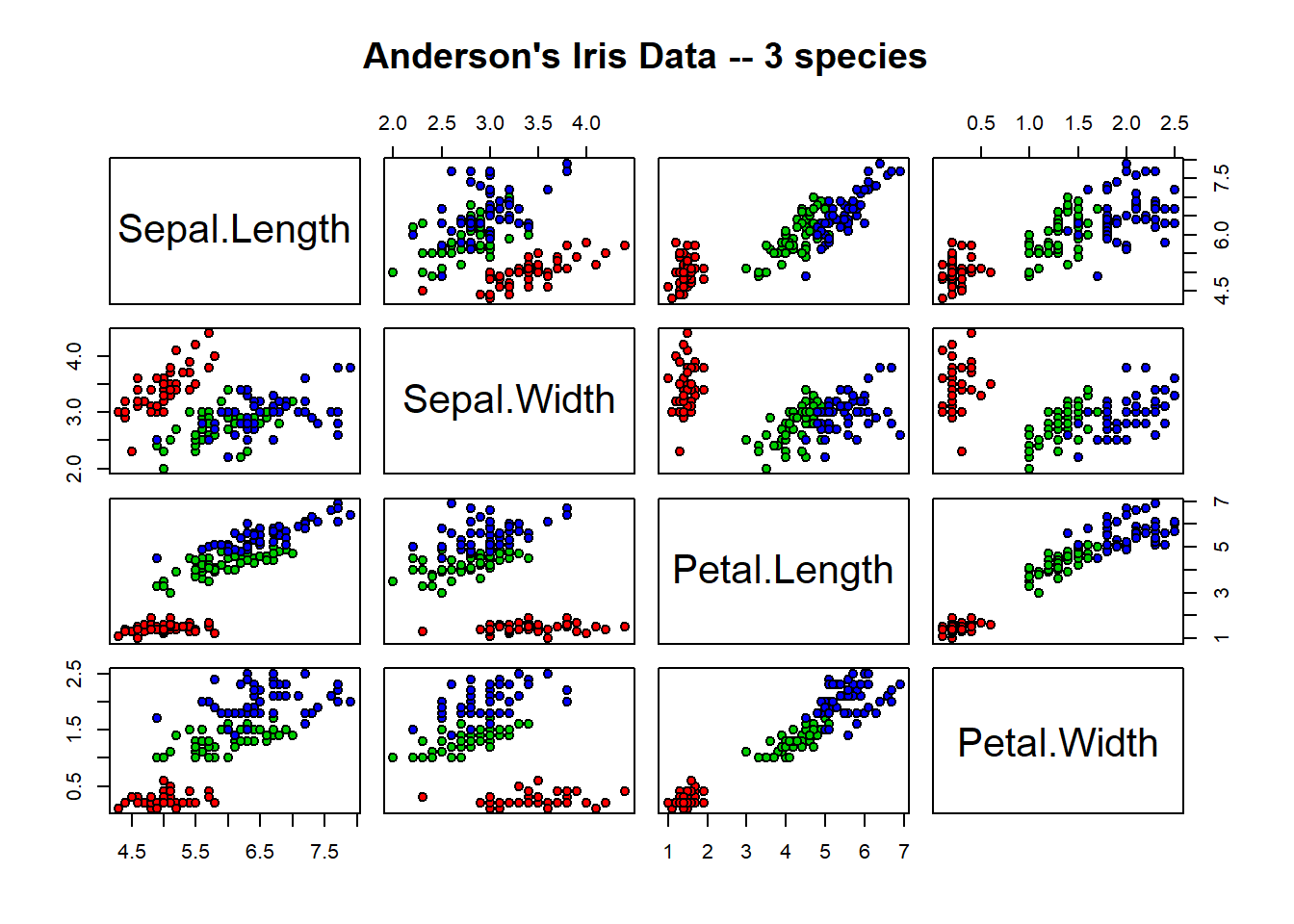``````## formula method, "graph" layout (row 1 at bottom):
pairs(~ Fertility + Education + Catholic, data = swiss, row1attop=FALSE,
subset = Education < 20, main = "Swiss data, Education < 20")``````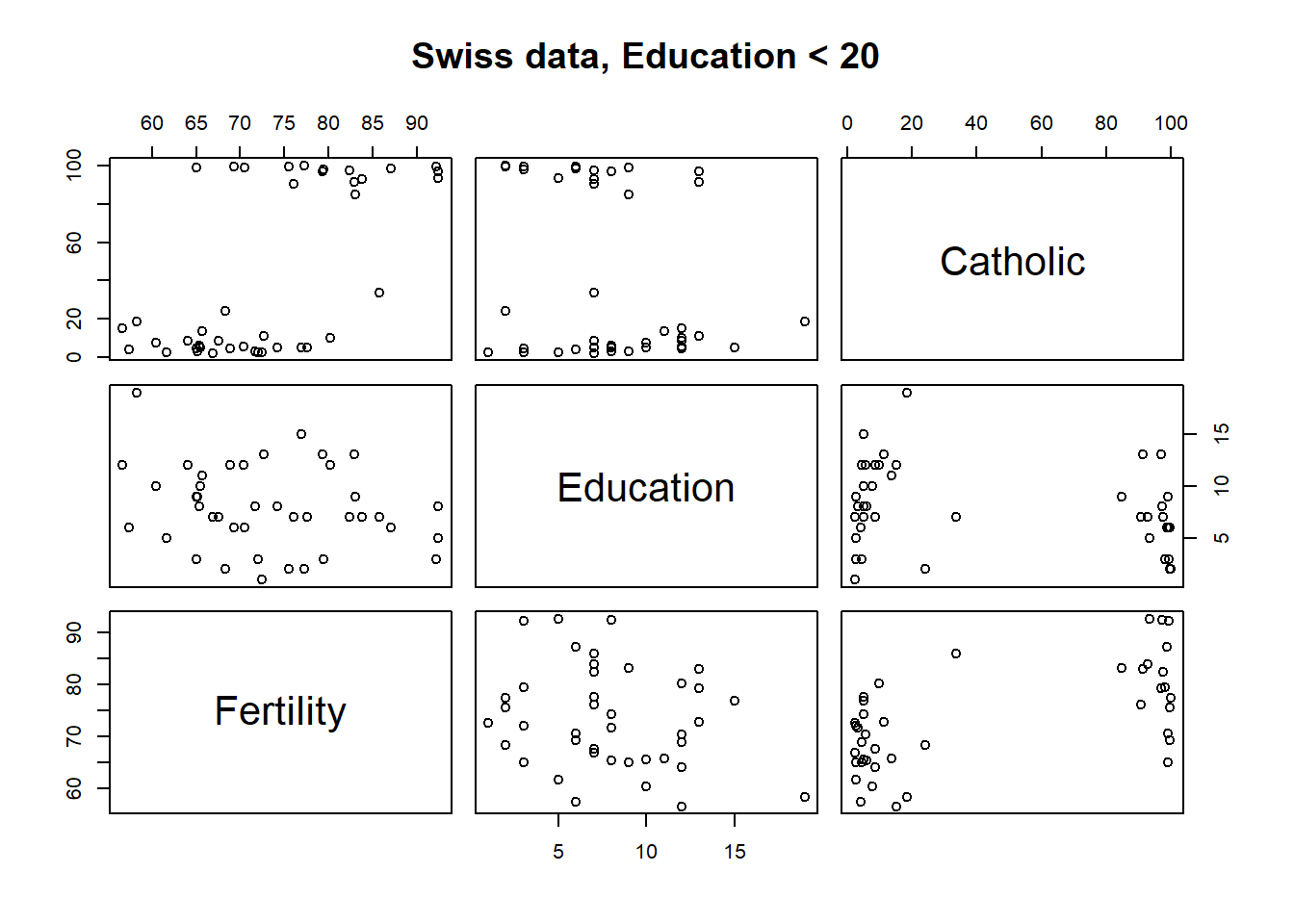``pairs(USJudgeRatings, gap=1/10) # (gap: not wasting plotting area)``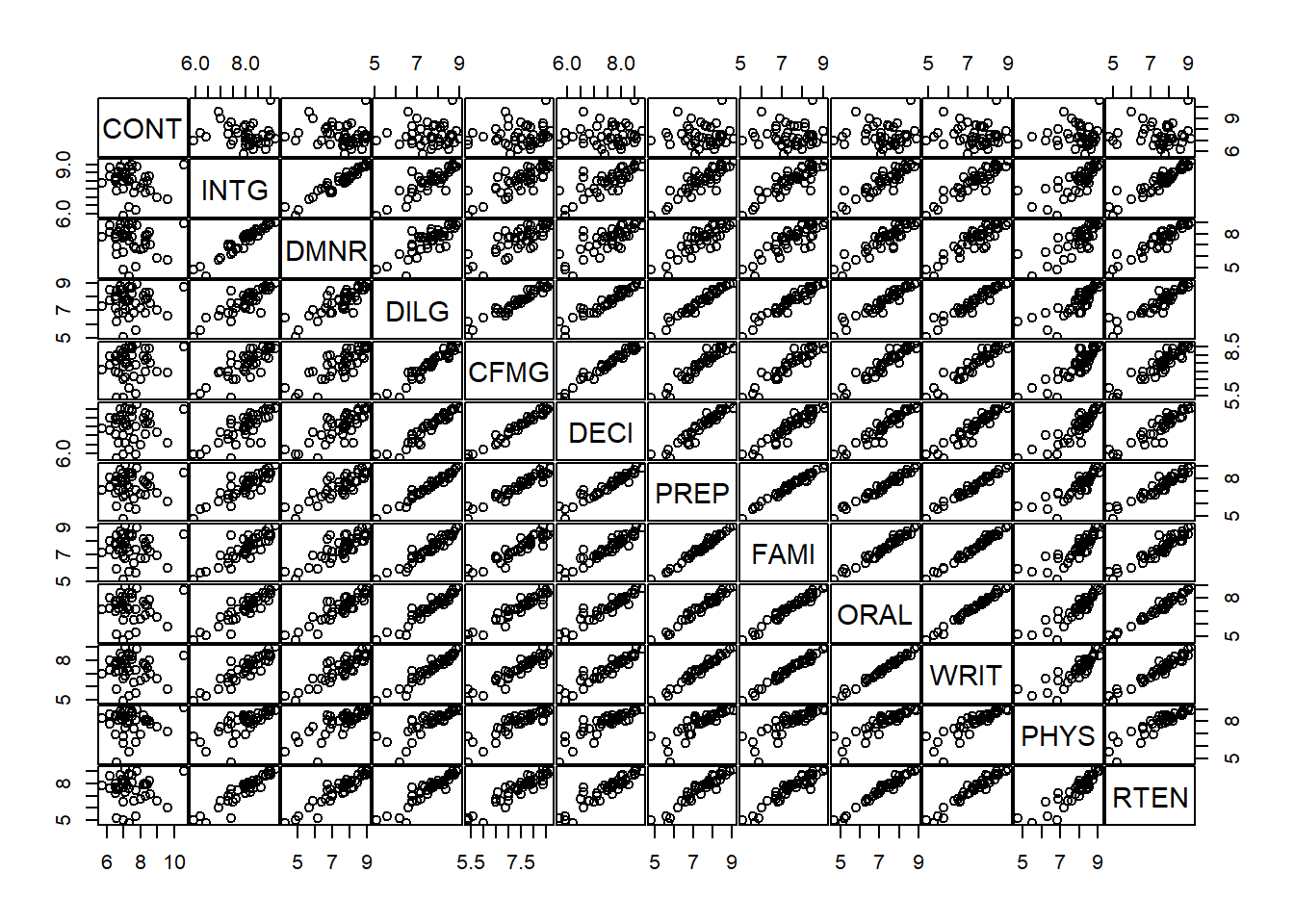``````## show only lower triangle (and suppress labeling for whatever reason):
pairs(USJudgeRatings, text.panel = NULL, upper.panel = NULL)``````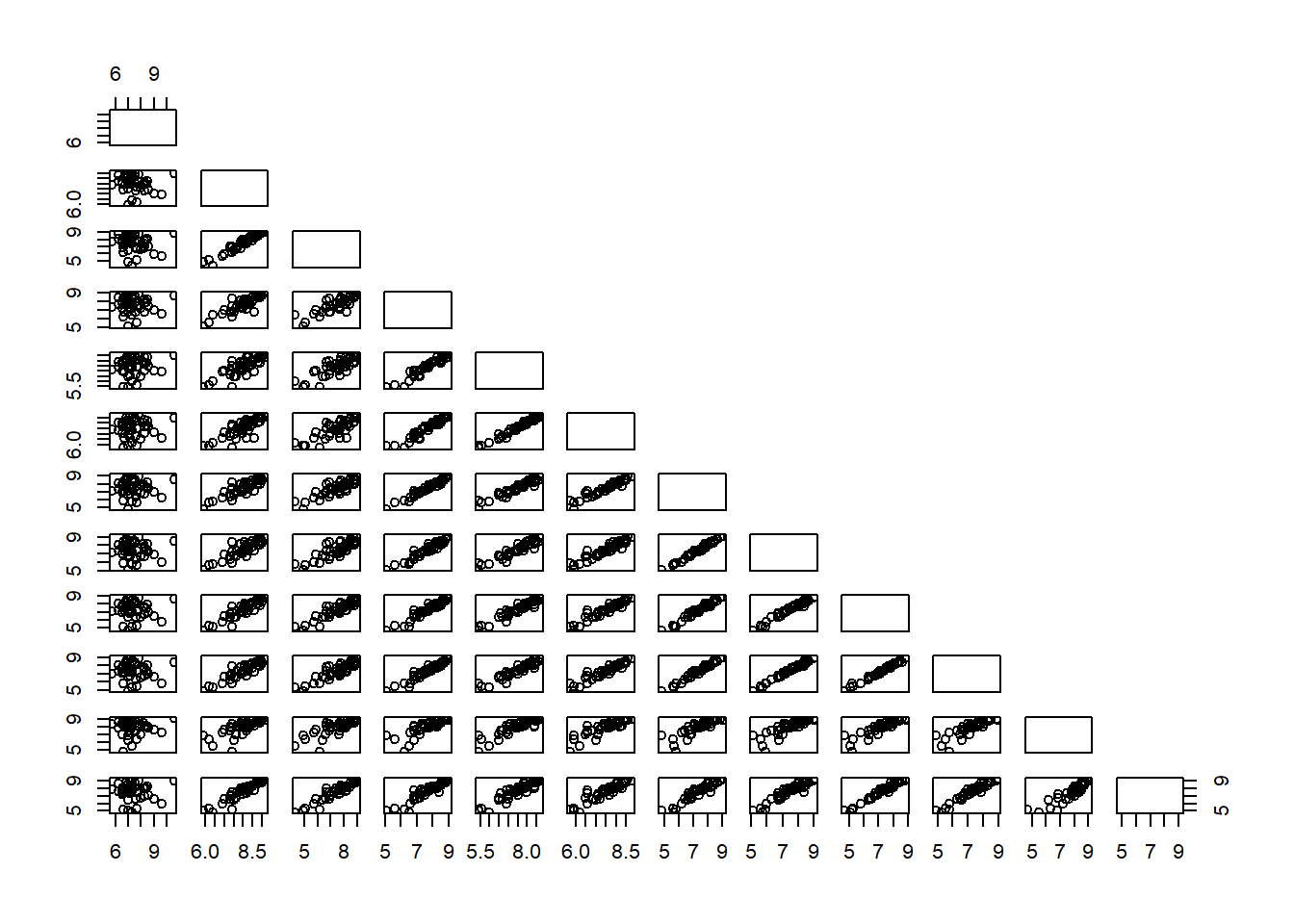``````## put histograms on the diagonal
panel.hist <- function(x, ...)
{
usr <- par("usr"); on.exit(par(usr))
par(usr = c(usr[1:2], 0, 1.5) )
h <- hist(x, plot = FALSE)
breaks <- h\$breaks; nB <- length(breaks)
y <- h\$counts; y <- y/max(y)
rect(breaks[-nB], 0, breaks[-1], y, col = "cyan", ...)
}
pairs(USJudgeRatings[1:5], panel = panel.smooth,
cex = 1.5, pch = 24, bg = "light blue", horOdd=TRUE,
diag.panel = panel.hist, cex.labels = 2, font.labels = 2)``````